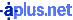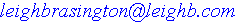## Mr. Worsham's Trigonometry Circle

I had a fantastic math teacher in high school. When I got to university, I was better prepared than any of my classmates - and that was all thanks to Mr. Harold Worsham's brilliant teaching. When he taught Trigonometry, he had this mnemonic circle to help with remembering how trig functions interact:
 tan sin sec cos csc cot
1. The inverse of any function is the one on the opposite side of the circle
e.g. the inverse of sin is csc, of cos is sec, and of tan is cot.

2. The product of 2 functions is the function between those 2 functions (assuming there is only one function between those 2;
if there are 2 between, the product is 1 (inverses!))
e.g. sin * sec = tan; tan * csc = sec; sec * cot = csc
e.g. csc * cos = cot; cot * sin = cos; cos * tan = sin
(and the product of 2 adjacent functions does not simplify.)

3. The result of dividing a function by one of its adjacent functions is the other adjacent function.
e.g. tan / sin = sec; sec / tan = csc; etc.

4. And obviously the "co" functions are directly above/below.

The mnemonic for remembering how to consctuct the circle is "c below."

 If you want to check any of the above, here's a reminder: h=Hypotenuse, a=Adjacent, & o=Opposite sin=o/h cos=a/h tan=o/a => (o/h) / (a/h) = sin/cos csc=h/o sec=h/a cot=a/o => (a/h) / (o/h) = cos/sin

Permalink http://leighb.com/trig.htm [] Hosted byLeigh Brasington // Revised 09 Aug 22